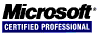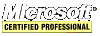# Intel IA-32 Assembly Tutorial

## Integer Arithmetic

This section covers bit shift and bit rotate operations. Because multiplication is a left bit shift operation and division is a right bit shift operation, multiplication and division are covered in this section.

All of the instructions in the table below affect the Overflow and Carry Flags.

 Shift and Rotate Instructions Mnemonic Description SHL shift left SHR shift right SAL shift arithmetic left SAR shift arithmetic right ROL rotate left ROR rotate right RCL rotate carry left RCR rotate carry right SHLD double-precision shift left SHRD double-precision shift right

#### Logical Shifts vs Arithmetic Shifts

A logical shift fills the newly created bit position with zero.

``````	mov al,10001111b
shl al,1         ;AL = 00011110b; CF = 1
mov al,10001111b
shr al,1         ;AL = 01000111b; CF = 1
mov al,11010000b
shr al,1         ;AL = 01101000b; CF = 0
``````

Fast, bit-wise multiplication using `SHL` is possible when you are multiplying by a base of 2. Shifting an unsigned integer n bits to the left multiplies it by 2n. For example, 8 is 23. Shift 3 to the left is multiply any number by 8.

``````	mov al,2         ;AL = 00000010b = 2 (02h)
shl al,3         ;AL = 00010000b = 16 (10h)
``````

Fast, bit-wise division using `SHR` is possible when you are dividing by a base of 2 operand. For example, 16 is 24. Shift 4 to the right is dividing any number by 16.

``````	mov al,32        ;AL = 00100000b = 32 (20h)
shr al,4         ;AL = 00000010b = 2 (02h)
``````

`SAL` (shift arithmetic left) is identical to `SHL`.

`SAR` can be used for signed division. `SAR` duplicates the sign bit.

``````	mov al,-128      ;AL = 10000000b = -128 (80h)
sar al,3         ;AL = 11110000b = -16 (F0h)
``````

The `ROL` instruction shifts each bit to the left and the highest bit is copied both into the Carry Flag and into the lowest bit. Bit rotation does not lose any bits like bit shifting does.

``````	mov al,40h       ;AL = 01000000b
rol al,1         ;AL = 10000000b; CF = 0
rol al,1		 ;AL = 00000001b; CF = 1
rol al,1		 ;AL = 00000010b; CF = 0
``````

Use `ROL` to exchange the upper bits with the lower bits.

``````	mov al,F1h
rol al,4         ;AL = 1Fh
``````

The `ROR` (rotate right) instruction shifts each bit to the right and copies the lowest bit into the Carry Flag and into the highest bit.

``````	mov al,1         ;AL = 00000001b
ror al,1         ;AL = 10000000b; CF = 1
ror al,1         ;AL = 01000000b; CF = 0
``````

The `RCL` (rotate carry left) instruction shifts each bit to the left, copies the Carry Flag into the least significant bit and copies the most significant bit into the Carry Flag.

``````	clc              ;CF = 0
mov bl,88h       ;CF = 0; BL = 10001000b (0 1000 1000)
rcl bl,1         ;CF = 1; BL = 00010000b (1 0001 0000)
rcl bl,1         ;CF = 0; BL = 00100000b (0 0010 0001)
``````

Recover a Bit from the Carry Flag: RCL can recover a bit that has previously been shifted into the Carry Flag.

``````	.data
byteval BYTE 01101010b
.code
shr byteval,1    ;shift least significant bit into Carry Flag
jc  quit_label   ;jump away if Carry Flag set
rcl byteval,1    ;otherwise, restore the number
``````

The `RCR` (rotate carry right) instruction shifts each bit to the right while copying the Carry Flag into the most significant bit and then copies the least significant bit into the Carry Flag.

``````	stc              ;CF = 1
mov ah,10h       ;CF = 1; AH = 00010000 (0001 0000 1)
rcr ah,1         ;CF = 0; AH = 10001000 (1000 1000 0)
``````

Shifting multiple doublewords:

``````	.data
ArraySize = 3
array DWORD ArraySize DUP (99999999h) ;9 = 1001b
.code
mov esi,0
shr array[esi+8],1                    ;high dword
rcr array[esi+4],1                    ;middle dword, include carry
rcr array[esi],1                      ;low dword, include carry
``````

The `MUL` (unsigned multiply) and `IMUL` (signed multiply) instructions multiply `EAX` by an operand. The product is stored in `EDX` and `EAX`. If, after the operation, EDX is not zero then the Carry Flag is set.

``````	mov eax,12345h
mov ebx,10000h
mul ebx          ;CF = 1, EDX:EAX = 00 00 00 01:23 45 00 00h
``````

The `DIV` instruction performs division on unsigned integers. A single operand is required and it must be either a register or memory operand. This operand is the divisor. The dividend is the `EDX:ECX` registers and the quotient is in the `EAX` register. The remainder is stored in `EDX`.

``````	.data
dividend QWORD 0000000800300020h
divisor	DWORD 00000100h
.code
mov edx,dword ptr dividend + 4 ;high dword
mov eax,dword ptr dividend     ;low dword
div divisor                    ;EAX = 08003000h, EDX = 00000020h
``````

Concerning signed integer division... These three instructions are needed. The `CBW` (convert byte to word) instruction extends the sign bit of `AL` into the `AH` register while preserving the number's sign. The `CWD` (convert word to doubleword) instruction extends the sign bit of the `AX` register into the `DX` register. The `CDQ` (convert doubleword to quadword) instruction extends the sign bit of the `EAX` register into the `EDX` register.

``````	.data
byteval SBYTE -65  ;9Bh
wordval SWORD -65  ;FF9Bh
dwrdval SDWORD -65 ;FFFFFF9Bh
.code
mov al,byteval
cbw                ;AX = FF9Bh
mov ax,wordval
cwd                ;DX:AX = FFFF:FF9Bh
mov eax,dwrdval
cdq                ;EDX:EAX = FFFFFFFF:FFFFFF9Bh
``````

The `IDIV` (signed divide) instruction performs signed integer division using the same operands as the `DIV` instruction, which requires that `EAX` be sign-extended into `EDX` using `CDQ` (convert doubleword into quadword).

``````	.data
dwrdval SDWORD -50000
.code
mov eax,dwrdval ;dividend low
cdq             ;extend EAX into EDX
mov ebx,256     ;divisor
idiv ebx        ;quotent EAX = -195; remainder EDX = -80
``````

If a divide operation has a quotient that is too large to fit into the destination operand then a divide overflow condition occurs. This and division by zero cause a CPU interrupt and the program halts.

<< < [Page 11 of 14] > >>